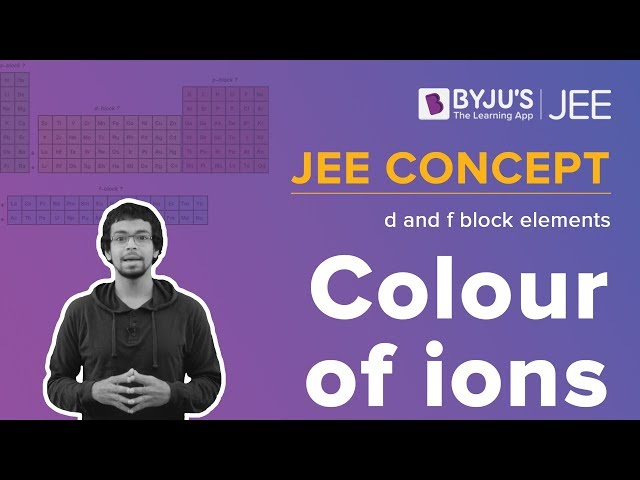Checkout JEE MAINS 2022 Question Paper Analysis : Checkout JEE MAINS 2022 Question Paper Analysis :

# d and f-Block Elements ‌–‌ ‌JEE‌ ‌Advanced‌ ‌Previous‌ ‌Year‌ ‌Questions with Solutions

d-and-f-Block Elements JEE Advanced Previous Year Questions with Solutions given here will allow JEE aspirants to study productively and boost their preparation for the entrance exam. We have provided a set of IIT JEE Advanced d-and-f-Block Elements past paper questions that are further accompanied by detailed step by step solutions and explanations. Going through the problems and solutions will help aspirants revise and at the same time understand the concepts more clearly. Additionally, they will also be able to get insight into the types of questions, the difficulty level and the overall pattern of the question paper. These will serve as a great last-minute preparation tool as well.

*Stay tuned as PDF will be added shortly.

## JEE Advanced Previous Year Questions on d-and-f-Block Elements

Question 1. Which of the following combination will produce H2 gas?

A. Fe Metal and conc. HNO3

B. Cu metal and conc. HNO3

C. Zn metal and NaOH(aq)

D. Au metal and NaCN(aq) in the presence of air

Solution: (C)

Zn metal and NaOH(aq) will produce hydrogen gas.

Zn + NaOH(aq) ⟶ Na2ZnO2 + H2

Question 2. The colour of light absorbed by an aqueous solution of CuSO4 is:

A. Orange-Red

B. Blue-Green

C. Yellow

D. Violet

Solution: (A)

The colour visible to the human eye is a complementary result of the colour absorbed.

Copper sulphate is blue in an aqueous solution. This means the complementary colour of blue is absorbed which we know to be closer to red. Thus, we can say that an orange-red wavelength is absorbed by the solution.

Question 3. An acidified solution of potassium chromate was layered with an equal volume of amyl alcohol. When it was shaken after the addition of 1 mL of 3% H2O2, a blue alcohol layer was obtained. The blue colour is due to the formation of a chromium (VI) compound ‘X’. What is the number of oxygen atoms bonded to chromium through only single bonds in a molecule of X?

Solution: (4)

The blue colour compound of ‘Cr’ is CrO5.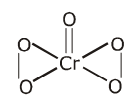Oxygen atoms bonded to chromium through only single bonds = 4

Question 4. In neutral or faintly alkaline solution, 8 moles of permanganate anion quantitative oxidize thiosulphate anions to produce X moles of sulphur-containing product. The magnitude of X is:

Solution: (6)

8MnO4 + 3S2O32- + H2O → 8MnO2 + 6SO42- + 2OH

Therefore, 8 moles of permanganate ion give 6 mols of sulphur-containing product.

Question 5. Consider the following list of reagent Acidified K2Cr2O7, alkaline KMnO4, CuSO4, H2O2, Cl2, O3, FeCl3, HNO3 and Na2S2O3 The total number of reagents that can oxidise aqueous iodide iodine is.

Solution: (7)

Acidified K2Cr2O7, CuSO4, H2O2, Cl2, O3, FeCl3, HNO3 oxidise aq. Iodide to iodine.

→ Alkaline KMnO4 oxidise aq. Iodide to IO3

→ No reaction between aq. Iodide and Na2S2O3

Reactions:

K2Cr2O7 + 7H2SO4 + 6KI → 4KrSO4 + Cr2(SO4)3 + 3I2 + 7H2O

2CuSO4 + 4KI → Cu2I2 + I2 + 2K2SO4

H2O2+ 2KI → 2KOH + I2

CP2 + 2KI → 2KCl + I2

O3 + 2KI + H2O → 2KOH + I2 + O2

2FeCl3 + 2KI → 2FeCl2 + I2 + 2KCl

2KMnO4 + KI + H2O → KIO3 + 2MnO2 + 2KOH

Na2S2O3 will not react with KI.

Question 6. Fusion of MnO2 along with KOH and O2 forms X. Electrolytic oxidation of X yields Y. X undergoes disproportionation reaction in acidic medium to MnO2 and Y. The manganese-containing ions present in W and X, respectively, are Y and Z. Correct statement(s) is(are).

A. Both Y and Z are coloured and have a tetrahedral shape

B. In an aqueous acidic solution, Y undergoes a disproportionation reaction to give Z and MnO2

C. Y is diamagnetic in nature while Z is paramagnetic

D. In both Y and Z, π-bonding occurs between p-orbitals of oxygen and d-orbitals of manganese

Solution: (A, B and D)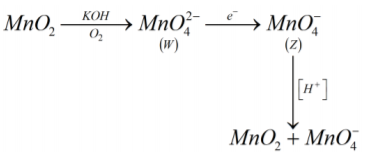Question 7. Consider the following reactions (unbalanced);

Zn + hot conc. H2SO4 → G + R + X

Zn + conc. NaOH → T + Q

G + H2S + NH4OH → Z (a precipitate) + X + Y Choose the correct option(s)

A. Z is dirty white in colour

B. Bond order of Q is 1 in its ground state

C. R is a V-shaped molecule

D. The oxidation state of Zn in T is + 1

Solution: (A, B and C)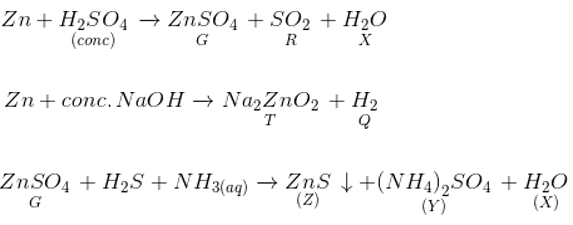A) SO2 is v-shaped.

B) ZnS is dirty white in colour.

C) Bond order of H2 is 1.

D) Oxidation state of Zn in Na2ZnO2 is +2.

Question 8. Fe3+ is reduced to Fe2+ by using

A. H2O2 in presence of NaOH

B. Na2O2 in water

C. H2O2 in presence of H2SO4

D. Na2O2 in presence of H2SO4

Solution: (A and B)

In basic medium H2O2 acts as a reducing agent.

Na2O2 + 2H2O → 2NaOH + H2O2

2Fe3+ + H2O2 + 2OH → 2Fe2+ + 2H2O + O2

Question 9. The correct statement(s) about Cr2+ and Mn3+ is (are) [Atomic numbers of Cr = 24 and Mn = 25]

A. Cr2+ is a reducing agent

B. Mn3+ is an oxidizing agent

C. Both Cr2+ and Mn3+ exhibit da electronic configuration.

D. When Cr2+ is used as a reducing agent, the chromium ion attains d5 electronic configuration.

Solution: (A, B and C)

Cr2+ is a reducing agent and Mn3+ is an oxidation agent and both have the electronic configuration d4.

Cr2+ loses electrons (and hence acts as a reducing agent) and forms higher oxidation states whereas Mn3+ gains electrons and thus acts as an oxidizing agent.

Question 10. The pair(s) of reagents that yield paramagnetic species is/are

A. Na and excess of NH3

B. K and excess of O2

C. Cu and dilute HNO3

D. O2 and 2-ethylanthraquinol

Solution: (A, B and C)

Dilute solution of Na in liquid ammonia is paramagnetic due to ammoniated e−1s

Na + (x + y)NH3 → Na + (NH3)x + e(NH3)y

K + O2 (excess) → KO2 (Paramagnetic)

O2 is paramagnetic due to unpaired electrons in antibonding orbital

3Cu + 8HNO3 (diluted) → 3Cu(NO3)2 + 2NO + 4H2O

NO is paramagnetic due to unpaired electrons on “N”.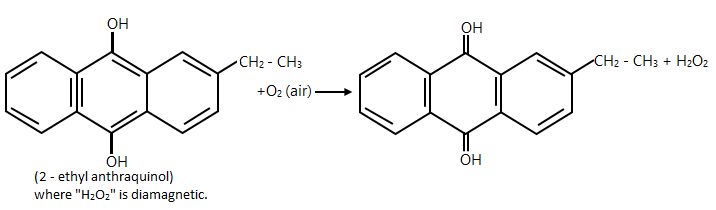## Alloy Formation of d & f-block elements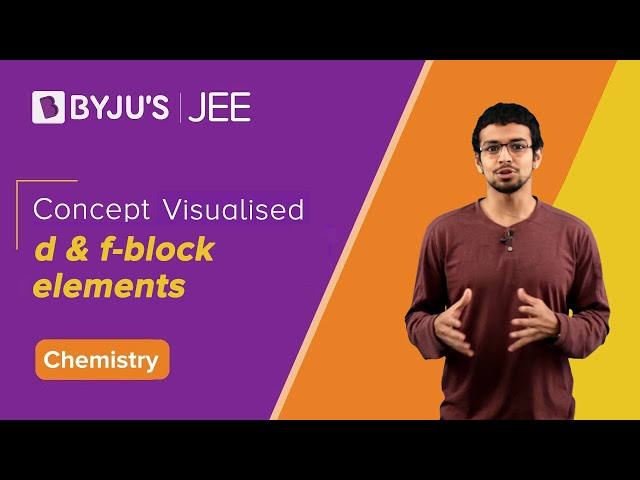## Colour of ions in d and f block elements